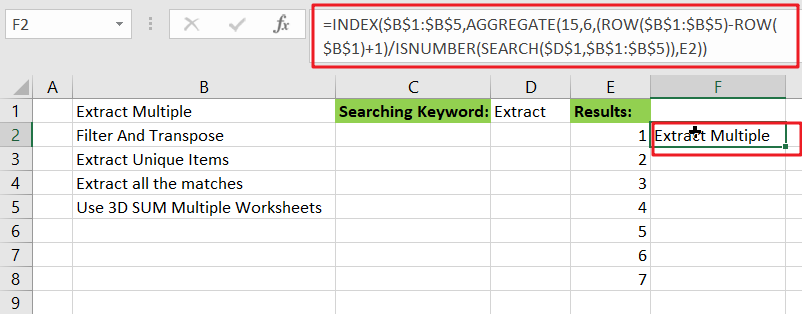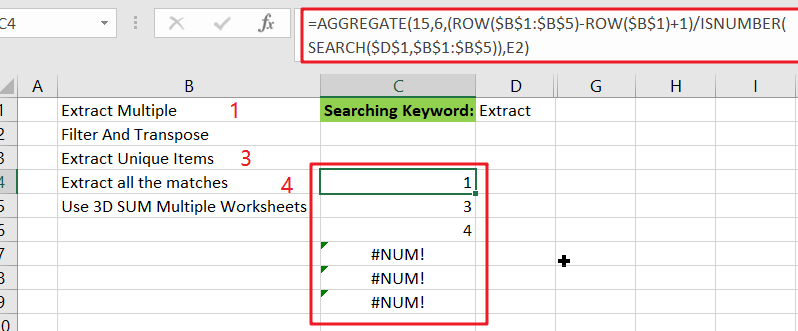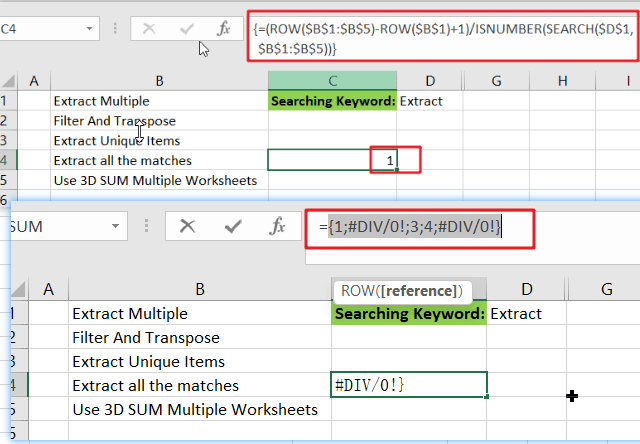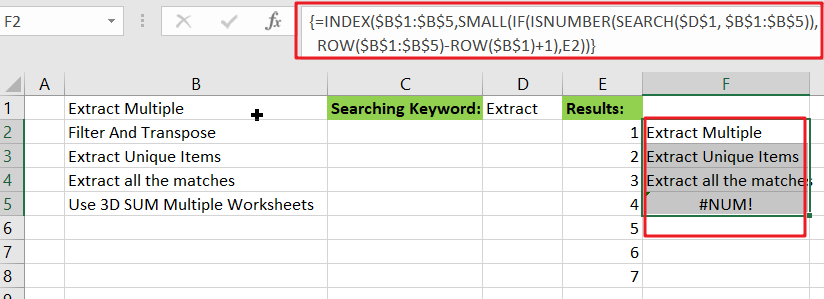# Extract all Partial Matches

Just assume that you have a range of data that contains a text string and you want to extract the few partial matches into another separate range of cells; then you might think that it’s not a big deal; because you would prefer to manually extract the few partial matches into another separate range of cells without any need of the formula;

Then congratulations because you are thinking right, but let me add up that it would be a big deal to extract the multiple partial matches into another separate range of cells and doing it manually would be a foolish attempt because you would get tired of it and would never complete your work on time.

But don’t be worry about it because after carefully reading this article, extracting multiple partial matches into another range of cells would become a piece of cake for you.

So let’s dive into the article to take you out of this fix.## General formula

The Following formula would help you out for extracting multiple partial matches into another separate range of cells:

`=INDEX(\$B\$1:\$B\$5,AGGREGATE(15,6,(ROW(\$B\$1:\$B\$5)-ROW(\$B\$1)+1)/ISNUMBER(SEARCH(\$D\$1,\$B\$1:\$B\$5)),E2))`

## Syntax Explanations

Before going into the explanation of the formula for getting the work done efficiently, we must understand each syntax which would make it easy for you that how each syntax contributes to extracting multiple partial matches into a separate range or list of cells:

• `INDEX`: In a range or array, this index function contributes to returning the value at a given position.
• `AGGREGATE`: This function contributes to returning the aggregate result in a database or list of values in the excel sheet.
• `ROW`: In Excel, this Row function contributes toreturning the row number as a reference.
• `Absolute Reference`: The Absolute referenceis nothing but an actual fixed location in a worksheet.
• `ISNUMBER`: This function contributes to returns TRUE when a cell contains a number and FALSE if there is no number.
• `SEARCH`: This function contributes to locating the character between two text strings and returns to the number of the starting position of the first text string from the first character of the second text string.
• `Comma symbol` (,): In Excel, this comma symbol acts as a separator that helps to separate a list of values.
• `Minus Operator` (-): This minus symbol contributes to subtracting any two values.
• `Parenthesis` (): The core purpose of this Parenthesis symbol is to group the elements and to separate them from the rest of the elements.
• `Plus operator` (+): This plus operator adds the values.
• `Division` (/): This division symbol is used for dividing values or numbers.

## Let’s See How This Formula Works:

The INDEX function is the primary function having AGGREGATE, which is highly useful to figure out the matches for each row in the extracted area:

`=INDEX(\$B\$1:\$B\$5,matched_values)`

Almost all of the work is to determine and report which rows in “`\$B\$1:\$B\$5`” match the search string and report the position of each matching value to INDEX. This is accomplished by configuring the AGGREGATE function as follows:

`=AGGREGATE(15,6,(ROW(\$B\$1:\$B\$5)-ROW(\$B\$1)+1)/ISNUMBER(SEARCH(\$D\$1,\$B\$1:\$B\$5)),E2)`The very first input or argument, which is `15`, instructs AGGREGATE to return the nth smallest values.

The second argument, `6`, specifies whether or not to ignore mistakes.

The third argument is an expression that returns an array of results that match.

The fourth input, `E2`, it specifies the “nth” value.

AGGREGATE works on arrays, and for the third argument inside AGGREGATE, the expression below builds an array :

`={(ROW(\$B\$1:\$B\$5)-ROW(\$B\$1)+1)/ISNUMBER(SEARCH(\$D\$1,\$B\$1:\$B\$5))}}`In this case, the ROW function is used to build an array of relative row numbers, as that’s why SEARCH and ISNUMBER are combined to match the search string against values in the data, resulting in an array of TRUE and FALSE values.

TRUE behaves as 1 in this math operation, while FALSE behaves as 0. As a result, row numbers with a positive match are divided by 1 and survive the operation, whereas row numbers with non-matching values are destroyed and become `#DIV/0 `errors. AGGREGATE is configured to ignore errors; it ignores #DIV/0 errors and returns the “nth” smallest number from the remaining values.

## Alternative Formula with SMALL Function

You can also use an alternative formula which is based on SMALL function and IF Function to achieve the same result:

`=INDEX(\$B\$1:\$B\$5,SMALL(IF(ISNUMBER(SEARCH(\$D\$1, \$B\$1:\$B\$5)),ROW(\$B\$1:\$B\$5)-ROW(\$B\$1)+1),E2))`Note: this is an array formula, and to get the work done, please enter it with `Control` `+` `Shift` `+` `Enter`.

### Related Functions

• Excel INDEX function
The Excel INDEX function returns a value from a table based on the index (row number and column number)The INDEX function is a build-in function in Microsoft Excel and it is categorized as a Lookup and Reference Function.The syntax of the INDEX function is as below:= INDEX (array, row_num,[column_num])…
• Excel ROW function
The Excel ROW function returns the row number of a cell reference.The ROW function is a build-in function in Microsoft Excel and it is categorized as a Lookup and Reference Function.The syntax of the ROW function is as below:= ROW ([reference])….
• Excel SMALL function
The Excel SMALL function returns the smallest numeric value from the numbers that you provided. Or returns the smallest value in the array.The syntax of the SMALL function is as below:=SMALL(array,nth) …
• Excel IF function
The Excel IF function perform a logical test to return one value if the condition is TRUE and return another value if the condition is FALSE. The IF function is a build-in function in Microsoft Excel and it is categorized as a Logical Function.The syntax of the IF function is as below:= IF (condition, [true_value], [false_value])….
• Excel ISNUMBER function
The Excel ISNUMBER function returns TRUE if the value in a cell is a numeric value, otherwise it will return FALSE.The syntax of the ISNUMBER function is as below:= ISNUMBER (value)…
• Excel SEARCH function
The Excel SEARCH function returns the number of the starting location of a substring in a text string.The syntax of the SEARCH function is as below:= SEARCH  (find_text, within_text,[start_num])…
• Excel AGGREGATE function
The Excel AGGREGATE function returns an aggregate in a list or database and ignore errors or hidden rows. The syntax of the AGGREGATE function is as below:= AGGREGATE(function_num, options, ref1,[ref2])…